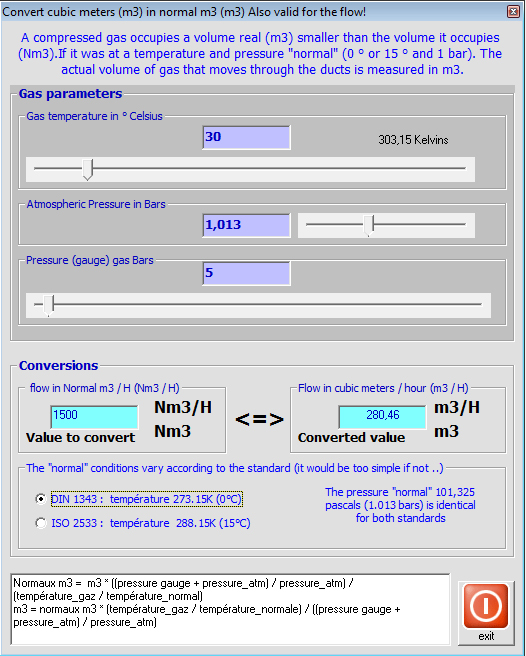The gas being compressible, it is possible to change a volume of the same amount of gasby compressing or changing its temperature. It becomes very difficult to speak of a quantity of gas volume without giving the pressure and temperature of the gas when the volume was measured. It would be difficult to give a temperature and pressure whenever we speak of a volume of gas. Then the temperature and pressure at the time of measurement of the volume, are normalized at called normal conditions. This is called normal m3.

Attention, there are two standards, and thus potential errors in conversions:

same pressure of 1013 millibars (average atmospheric pressure) for 2 standards:

DIN 1343 : temperature 273.15K (0°C)
ISO 2533 : temperature 288.15K (15°C)

For calculations of head loss, the size of volumes of gas (compressed or not) moved into the ducts must be given in cubic meters (m3).

For conversions or Nm3 Nm3 m3 / h m3 / h:

P1V1/T1 = P2V2/T2

p1 and T1 being normal temperatures and pressures v1 is the normal volume (relaxed)

p2 and T2 being the temperature and pressure gas V2 is the volume of the compressed gas

The converter integrated in Mecaflux Standard

The conversion interface normal volume (nm3) and real (m3) converts the normal flow (Nm3 / h) to real (m3 / h)